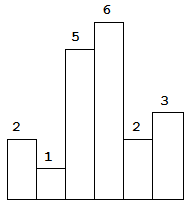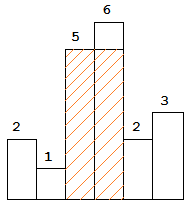## Largest Rectangle in Histogram

06/28/2016 Array Stack

## Question

Given n non-negative integers representing the histogram’s bar height where the width of each bar is 1, find the area of largest rectangle in the histogram.Above is a histogram where width of each bar is 1, given height = [2,1,5,6,2,3].The largest rectangle is shown in the shaded area, which has area = 10 unit.

For example,

Given heights = [2,1,5,6,2,3], return 10.

## Solution

Result: Accepted Time: 8 ms

Here should be some explanations.

int stck;
int largestRectangleArea(int* hgt, int hgtsz) {
int top = 0,ret = 0;
for(int i = 0; i <= hgtsz; i ++)
{
int now = (i == hgtsz) ? 0:hgt[i];
while(top && now < hgt[stck[top-1]])
{
int h = hgt[stck[--top]];
int width = (top?i-1 - stck[top-1] : i);
int tmp = h*width;
if(tmp > ret)
ret  = tmp;
}
stck[top++] = i;
}
return ret;
}


Complexity Analytics

• Time Complexity: $O(n)$
• Space Complexity: $O(n)$